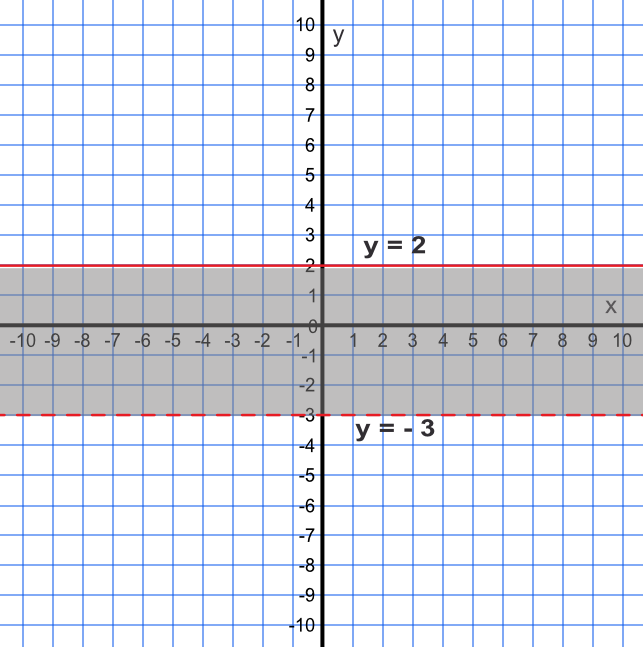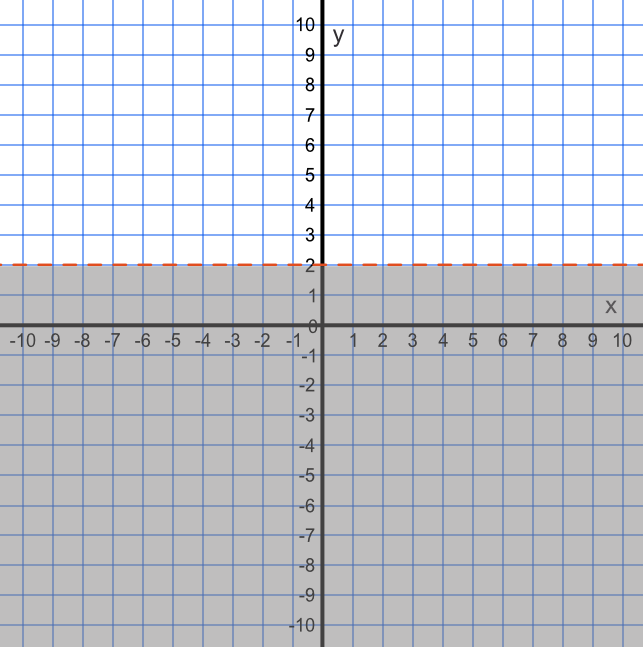Inequalities on a Graph

## Inequalities on a Graph

Inequalities can be represented on a graph. The inequality is shown by the line of the inequality, and a shaded area. The line of the inequality is shown in one of two ways:

• a solid line indicates that the line is included in the inequality: it is either ≤ or ≥;

• a dotted line indicates that the line is not included: it is either > or < .

## Example 1

Shade, on a graph, the inequality described by -3 < y ≤ 2.

Note that the line y = 2 is a solid line (≤), and that y = -3 is a dotted line## Example 2

What inequality does the graph, represented by the shading and the line, describe?

Write the inequality using set notation.The shaded area is less than 2: because the line is dotted, the value of y = 2 is not included.

Answer: {y: y < 2}